# Node-Red Function Node Multiple Outputs (How to Use Function Node)

In this tutorial, we will examine the multi-message output (Outputs) case of the function node in the red node. In this example we will create a function with 3 outputs. You can create as much as you need.

## 1)Let’s add Node-Red Function Outputs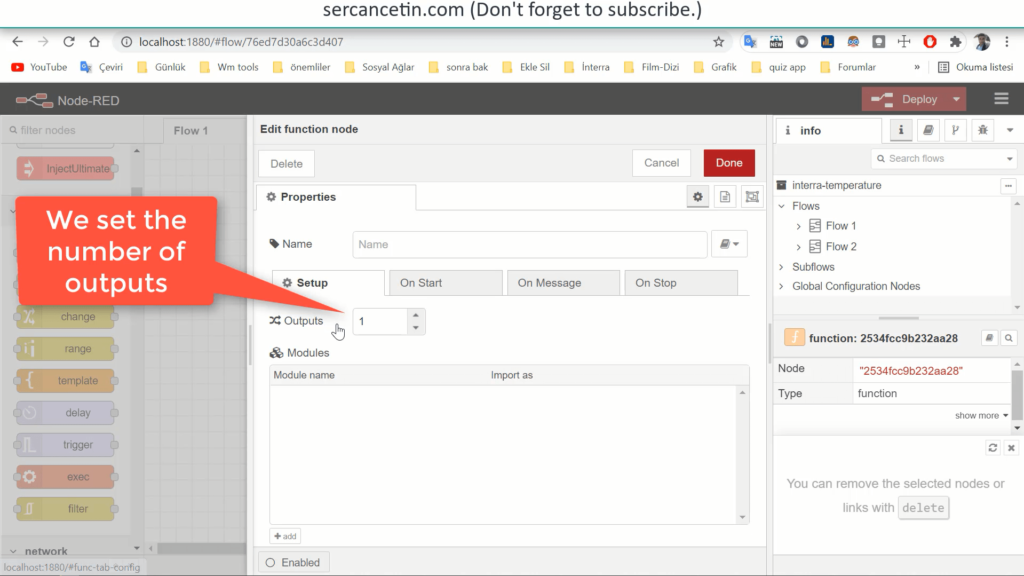From the “Properties” settings of the function to the “Installation” section. Let’s set the entry in the “Outputs” section to 3.

## 2) We are creating the data that will go to the Outputs.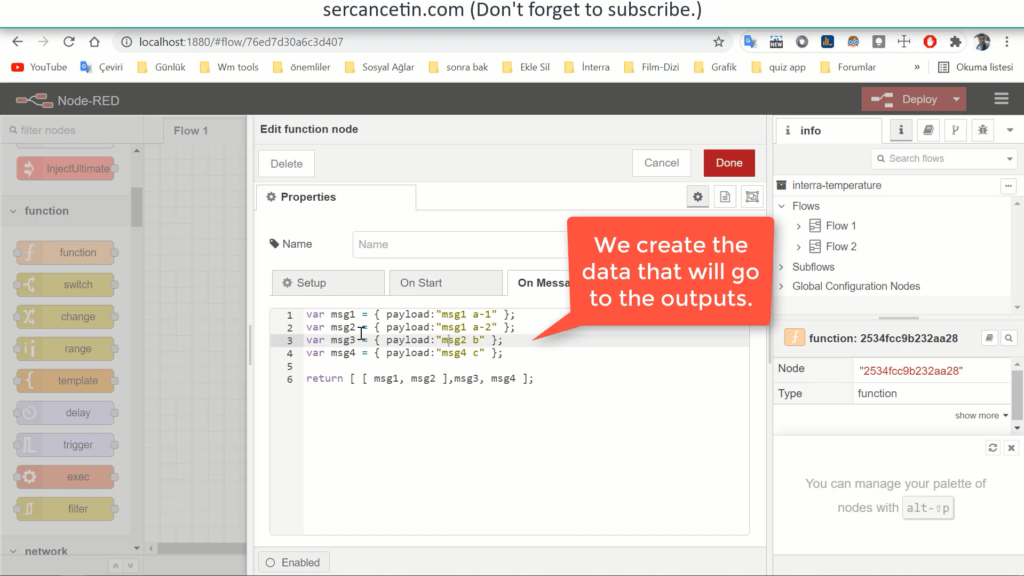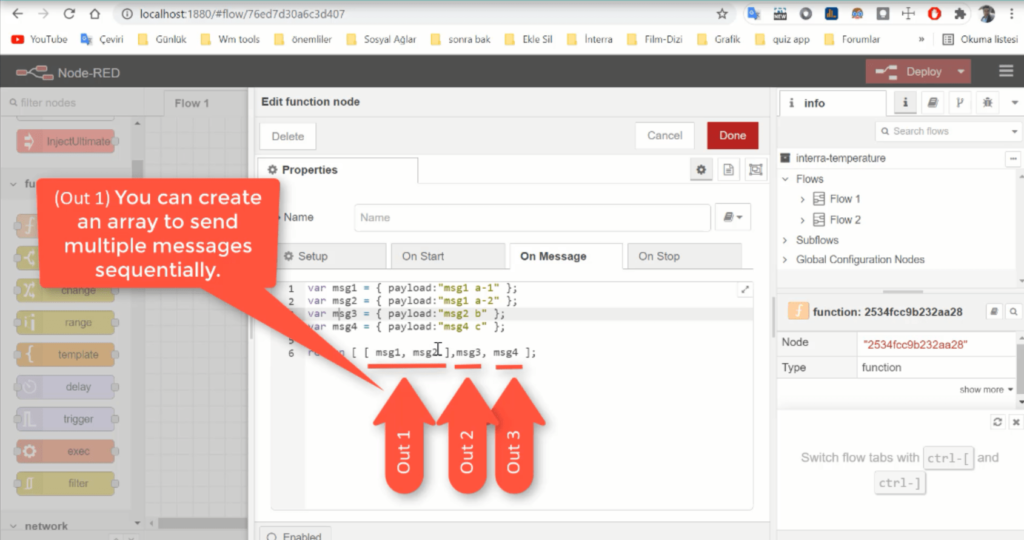You can create an array to send multiple messages consecutively.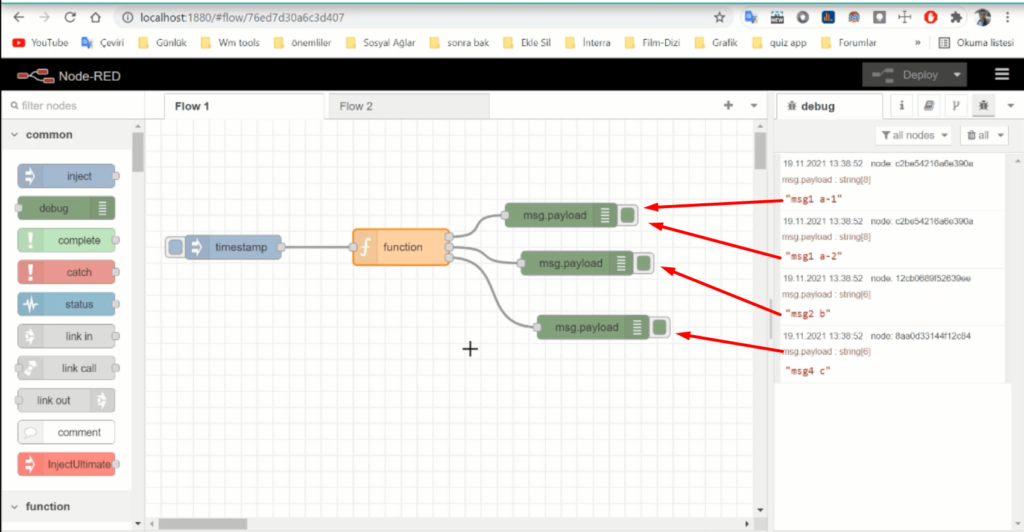```	1.Output = msg1,msg2
2.Output = msg3
3.Output = msg4
```

## Let’s create a different scenarioSuppose a json data is received.(Line 3) We are converting String data to object.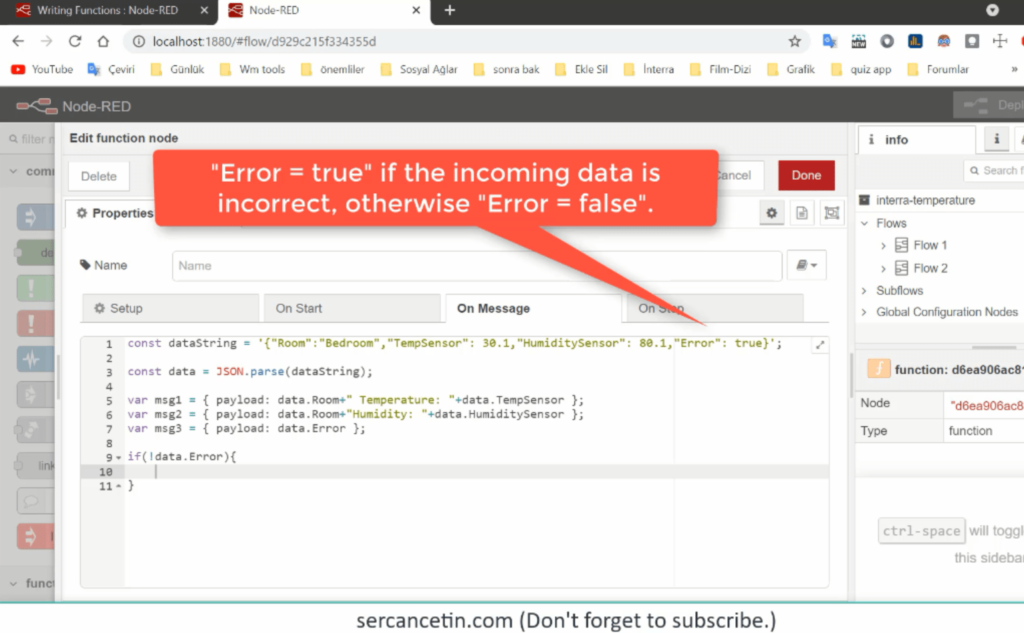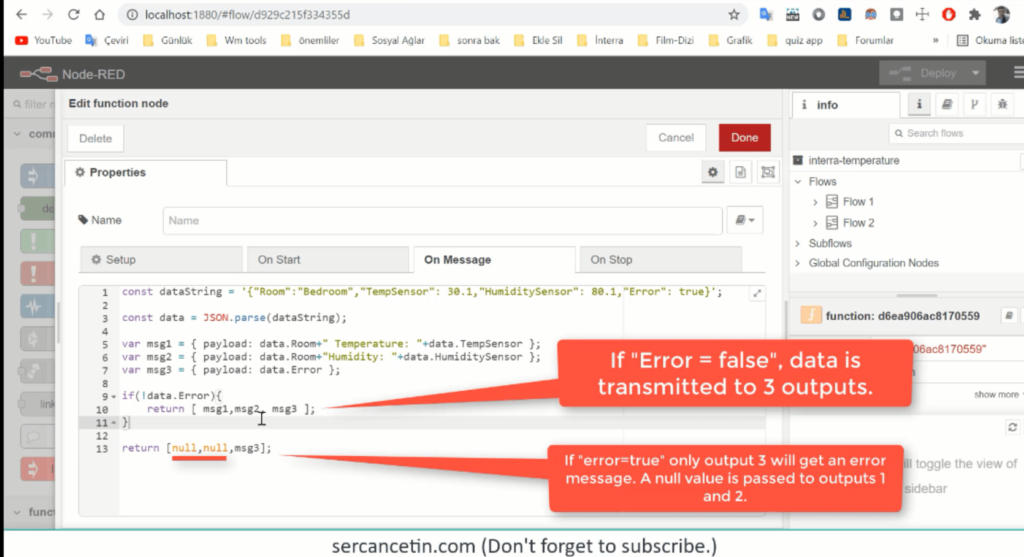(Line 9) We check for incorrect data from the sensor.
“Error = true”, “Error = false” if the incoming is false.If “Error = false”, data is transmitted to 3 outputs.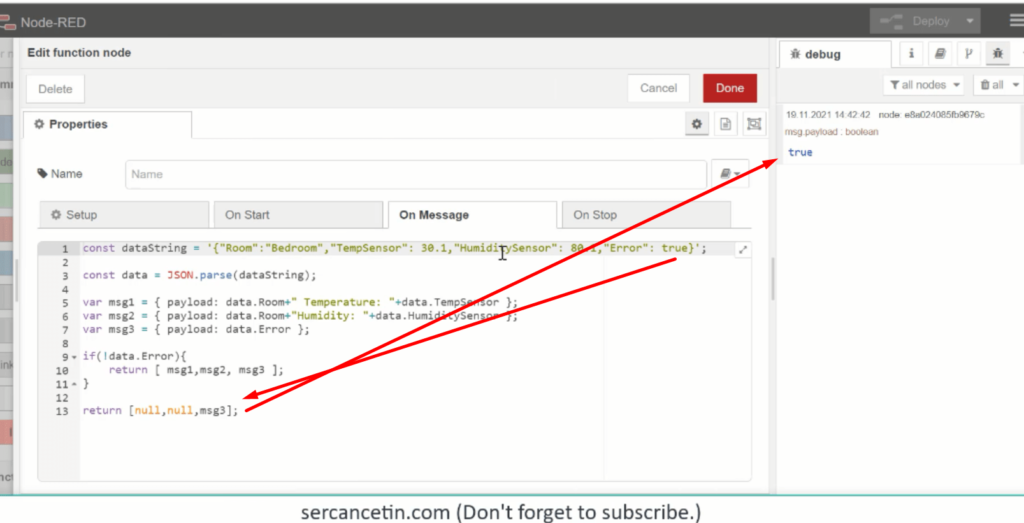If “Error = true”, only output 3 will get an error message. A null value is passed to outputs 1 and 2.

This site uses Akismet to reduce spam. Learn how your comment data is processed.